# The Minimum Spanning Tree (MST) problem

## Overview

The Minimum Spanning Tree (MST) problem is a classic computer science problem.

We will study the development of algorithmic ideas for this problem, culminating with Chazelle's O(m α(m,n))-time algorithm, an algorithm that easily meets the "extreme" criterion.

A preview:

• How is the MST problem defined?

• Simple algorithms: Kruskal and Jarnik-Prim.

• A generalization from the past: Boruvka.

• The basic binary heap and its descendants.

• A randomized algorithm.

• Chazelle's and Pettie's (independently discovered) algorithms.

Our presentation will pull together material from various sources - see the references below. But most of it will come from [Eisn1997], [Pett1999], [CS153], [Weis2007].

## Background: what is a graph?

Informal definition:

• A graph is a mathematical abstraction used to represent "connectivity information".

• A graph consists of vertices and edges that connect them, e.g.,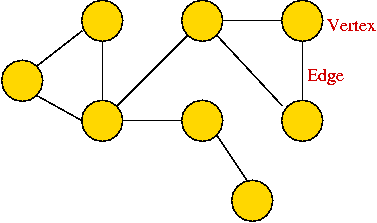• It shouldn't be confused with the "bar-chart" or "curve" type of graph.

Formally:

• A graph G = (V, E) is:
• a set of vertices V
• and a set of edges E = { (u, v): u and v are vertices }.

• Two types of graphs:
• Undirected graphs: the edges have no direction.
• Directed graphs: the edges have direction.

• Example: undirected graph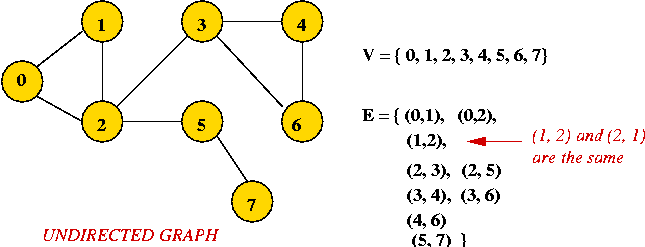• Edges have no direction.
• If an edge connects vertices 1 and 2, either convention can be used:
• No duplication: only one of (1, 2) or (2, 1) is allowed in E.
• Full duplication: both (1, 2) and (2, 1) should be in E.

• Example: directed graph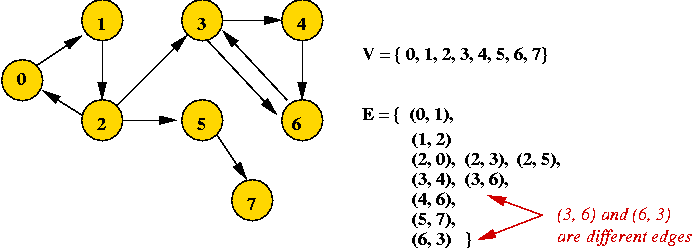• Edges have direction (shown by arrows).
• The edge (3, 6) is not the same as the edge (6, 3) (both exist above).

• For the MST problem: we will only use undirected graphs.

Depicting a graph:

• The picture with circles (vertices) and lines (edges) is only a depiction
a graph is purely a mathematical abstraction.

• Vertex labels:
• Can use letters, numbers or anything else.
• Convention: use integers starting from 0.
useful in programming, e.g. degree[i] = degree of vertex i.

• Edges can be drawn "straight" or "curved".

• The geometry of drawing has no particular meaning: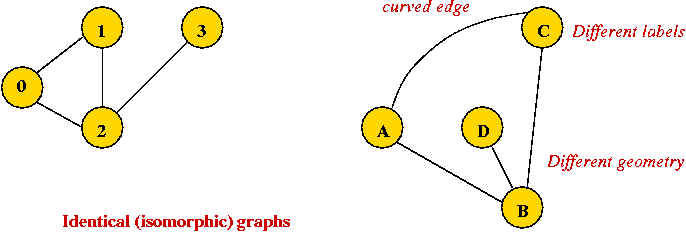Graph conventions:

• What's allowed (but unusual) in graphs:
• Self-loops (occasionally used).
• Multiple edges between a pair of vertices (rare).
• Disconnected pieces (frequent in some applications).
Example: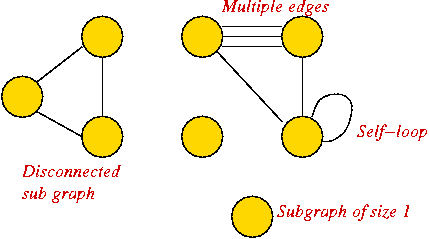• What's not (conventionally) allowed:
• Mixing undirected and directed edges.
• Re-using labels in vertices.
• Bidirectional arrows.
Exercise: If we disallow multiple edges and self-loops, what is the maximum number of edges in an undirected graph with n vertices? What is this number in order-notation?

Definitions (for undirected graphs):

• Degree of a vertex: number of edges incident to it.

• Neighbors: Two vertices are neighbors (or are adjacent) if there's an edge between them.

• Paths:
• Path: a sequence of vertices in which successive vertices are adjacent.
• A simple path does not repeat any vertices (and therefore edges) in the sequence.
• A cycle is a simple path with the same vertex as the first and last vertex in the sequence.

• Connectivity: two vertices are connected if there is a path that includes them. Weighted graph:
• Sometimes, we include a "weight" (number) with each edge.
• Weight can signify length (for a geometric application) or "importance".
• Example: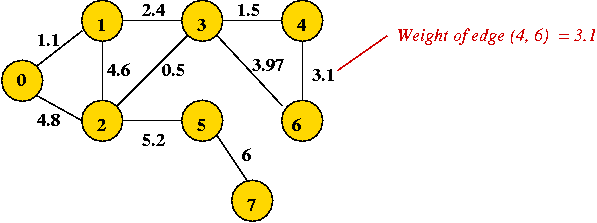Euclidean graph:
• The vertices are points in the plane.
• Edges are implied (all pairs) with Euclidean distance as weights.
• Note: one can define a version for d-dimensions.
Trees:
• A tree is a connected graph with no cycles.

• A spanning tree of a graph is a connected subgraph that is a tree.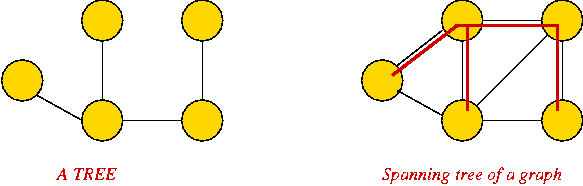Data structures for graphs:

• Key idea: use a 2D matrix.
• Row i has "neighbor" information about vertex i.
• Here, adjMatrix[i][j] = 1 if and only if there's an edge between vertices i and j.
• Example: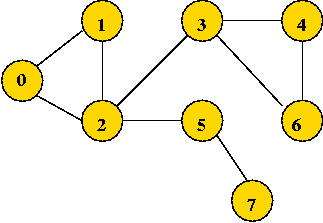```
0 1 1 0 0 0 0 0
1 0 1 0 0 0 0 0
1 1 0 1 0 1 0 0
0 0 1 0 1 0 1 0
0 0 0 1 0 0 1 0
0 0 1 0 0 0 1 1
0 0 0 1 1 1 0 0
0 0 0 0 0 1 0 0
```

• Key idea: use an array of vertex-lists.
• Each vertex list is a list of neighbors.
• Example: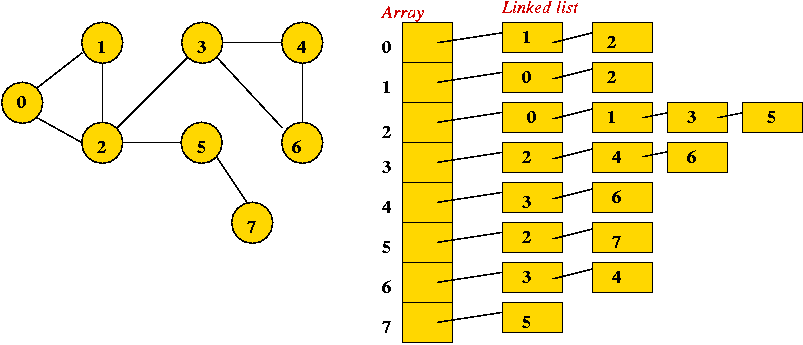• Convention: in each list, keep vertices in order of insertion

• Both representations allow complete construction of the graph.

• Simple to program.
• Some matrix operations (multiplication) are useful in some applications (connectivity).
• Efficient for dense (lots of edges) graphs.

• Less storage for sparse (few edges) graphs.
• Easy to store additional information in the data structure.
(e.g., vertex degree, edge weight)

• Implementations for MST use adjacency lists.

## Defining the MST problem

With this background, it's going to be easy to state the MST problem: find the spanning tree of minimum weight (among spanning trees):

• Input: a weighted, connected graph.

• Output: find a sub-graph such that
• The sub-graph is a connected tree.
• The tree contains all graph vertices.
it's a spanning tree.
• The tree weight is defined as the sum of edge-weights in the tree.
• The tree weight is the least among such spanning trees.

Exercise:

• "Eyeball" the weighted graph below and find the minimum spanning tree, and the shortest path from vertex 0 to vertex 6.• Try the example given to you in class.

Some assumptions and notation for the remainder:

• Let n = |V| = number of vertices.
• Let m = |E| = number of edges.
• We will assume unique edge weights. If this is not true, some arbitrary tie-breaking rule can be applied.
It simplifies analysis.

## Minimum Spanning Trees: Two Key Observations

The Cut-Edge Observation:

• Consider a partition of the vertices into two sets.

• Suppose we re-draw the graph to highlight edges going from one set to the other: (without changing the graph itself)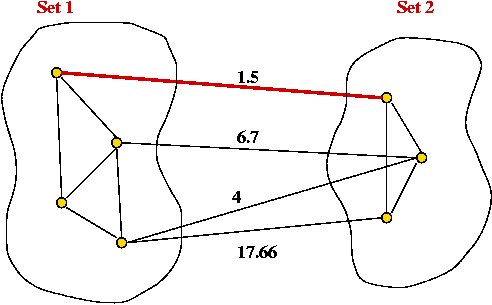• Cut-edge: an edge between the two sets.

• Consider the minimum-weight edge between the sets.
(Assume the edge is unique, for now).

• The minimum-weight edge must be in the minimum spanning tree.

Exercise: Why?

Prim's algorithm (a preview):

• At each step, find an edge (cut-edge) of minimum-weight between the two sets.

• Add this edge to MST and move endpoint from Set2 into Set1 .

• Repeat until Set1 = {all vertices}.

The Cycle Property:

• First, note: adding an edge whose end-points are in the MST will always cause a cycle.

• Consider adding an edge that causes a cycle in current MST.

• Suppose the edge has weight larger than all edges in cycle
no need to consider adding edge to MST.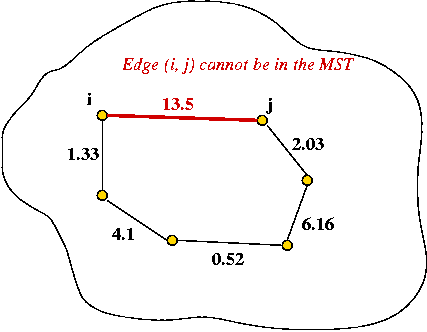• Therefore, if edges are added in order of (increasing) weight
no need to consider edges that cause a cycle.

Kruskal's algorithm (a preview):

• Sort edges in order of increasing weight.
• Process edges in sort-order.
• For each edge, add it to the MST if it does not cause a cycle.

## Kruskal's Algorithm

Key ideas:

• Sort edges by (increasing) weight.

• Initially, each vertex is a solitary sub-graph (MST-component).

• Start with small MST-component's and grow them into one large MST.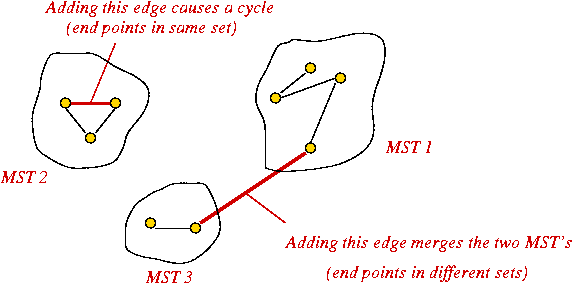• Need to track: which vertex is in which component (i.e., set).

Exercise: Show the steps in Kruskal's algorithm for this example:Implementation:

• High-level pseudocode:
```
Algorithm: Kruskal-MST (G)
Input: Graph G=(V,E) with edge-weights.

1.   Initialize MST to be empty;
2.   Place each vertex in its own set;
3.   Sort edges of G in increasing-order;
4.   for each edge e = (u,v) in order
5.       if u and v are not in the same set
7.           Compute the union of the two sets;
8.       endif
9.   endfor
10.  return MST

Output: A minimum spanning tree for the graph G.
```

• Now, let's use a union-find algorithm and explain in more detail:
```

1.   Initialize MST to be empty;

// Initialize union-find algorithm and
// place each vertex in its own set.
2.   for i=0 to numVertices-1
3.       makeSingleElementSet (i)
4.   endfor

5.   sortedEdgeList = sort edges in increasing-order;

6.   Initialize treeMatrix to store MST;

// Process edges in order.
7.   while sortedEdgeList.notEmpty()
8.       edge (i,j) = extract edge from sortedEdgeList.
// If edge does not cause a cycle, add it.
9.       if find(i) != find(j)
10.          union (i, j)
11.          treeMatrix[i][j] = treeMatrix[j][i] = adjMatrix[i][j]
12.      endif
13.  endwhile

14.  return treeMatrix

Output: adjacency matrix represention of tree.
```

• To place edges in list: simply scan each vertex list once.
O(m) time.

• Sorting: O(m log(m)).

• O(log(n)) per union or find.

• Total: O(m)+ O(m log(m)) + O(m log(n))
O(m log(m))
O(m log(n))

## Prim's Algorithm

Key ideas:

• Start with vertex 0 and set current MST to vertex 0.

• At each step, find lowest-weight edge from an MST vertex to a non-MST vertex and add it to MST.

• To record the weight of each edge, we will associate edge weight with the vertex that's outside the MST.

• We will associate a "priority" with each vertex:
• Priority(v) is defined only for v not in current MST.
• Intuitively, priority(v) = cost of getting from current MST to v.
• For example, consider node A with priority(A) = 3.1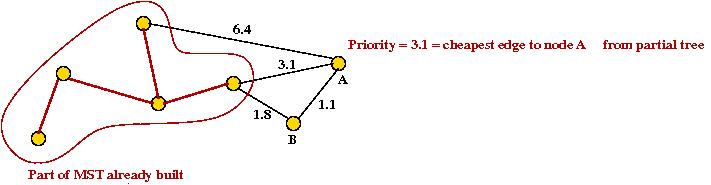• priority(A) changes to 1.1 after B is added.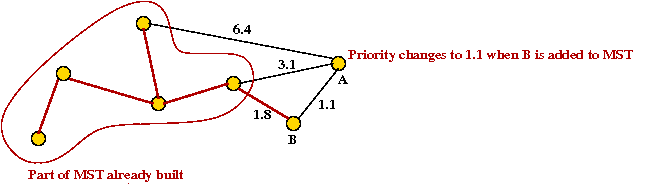Example:

• Initially, place vertex 0 in the MST and set the "priority" of each vertex to infinity.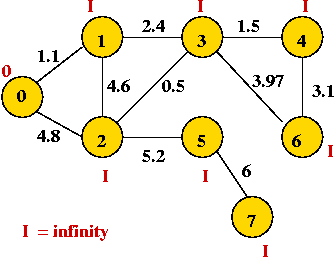• Explore edges from current MST: (0, 1) and (0, 2).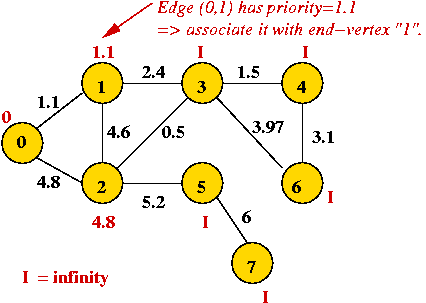• Pick lowest-weight edge (0, 1) to add
same as selecting lowest-priority vertex (vertex 1)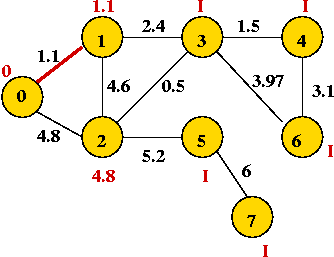• Explore edges from newly-added vertex: (1,3), (1,2)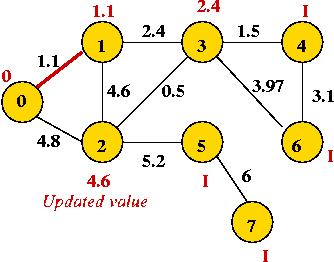That's why we associate edge-weights with vertices
only the edges from the new vertex add new information at each step.

• Pick vertex with lowest priority (vertex 3) and explore its edges: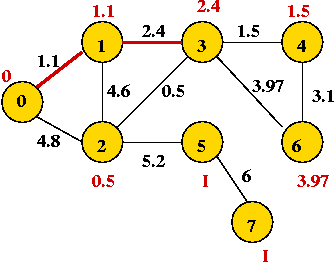• Continuing, we add vertices 2, 4, 6, 5 and 7: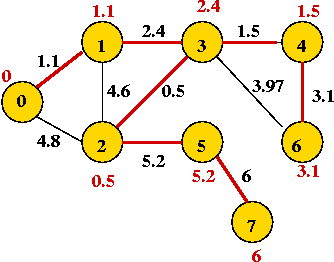Implementation:

• High-level pseudocode:
```
Algorithm: Prim-MST (G)
Input: Graph G=(V,E) with edge-weights.

1.   Initialize MST to vertex 0.
2.   priority = 0
3.   For all other vertices, set priority[i] = infinity
4.   Initialize prioritySet to all vertices;
5.   while prioritySet.notEmpty()
6.       v = remove minimal-priority vertex from prioritySet;
7.       for each neighbor u of v
9.           w = weight of edge (v, u)
8.           if w < priority[u]
9.               priority[u] = w        // Decrease the priority.
10.          endif
11.      endfor
12.  endwhile

Output: A minimum spanning tree of the graph G.
```

Exercise: Consider line 6 in the algorithm above. How long does it take to execute? Analysis:

• Each edge is added once to the priority set.

• There are O(n) removals.

• Since each edge is processed once, there are at most O(m) priority adjustments.

• Thus, if a removal takes time r(n) and and a priority-decrease takes time d(n)
O(n r(n) + m d(n)) time overall.

Exercise: What is the running time when using a list for the priority set?

• A sorted list.
• An unsorted list.

## Enter the data structures, part I: the binary heap

What is a priority queue?

• Keys or key-value pairs are stored in a data structure.

• The operations usually supported are:
• Insert: insert a new key-value pair.
• Delete: delete a key-value pair.
• Search: given a key, find the corresponding value.
• Find minimum, maximum among the keys.

A priority queue with the decreaseKey operation:

• For convenience, we will combine minimum and remove (delete) into a single operation: extractMin
• We would like to change a key while it's in the data structure
location in data structure also needs to be changed.

• Potentially O(n) time for decreaseKey
• O(1) time for extractMin

• Unsorted array:
• Potentially O(n) time for extractMin
• O(1) time for decreaseKey

• Can we do better?
• O(log(n)) time for extractMin?
• O(log(n)) time for decreaseKey?

Implementing a priority queue with a binary heap:

• Recall extractMin operation (Module 2):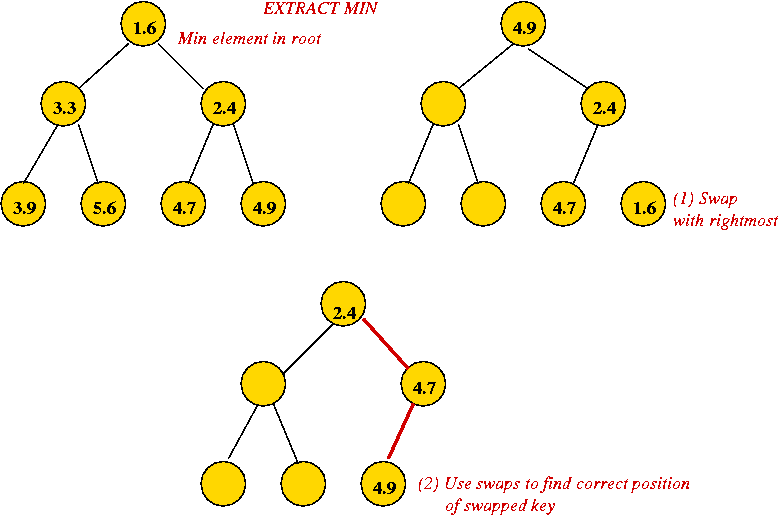• Height of heap is O(log(n)) always.
• Each extractMin results in changes along one tree path.
O(log(n)) worst-case.

• Implementing decreaseKey: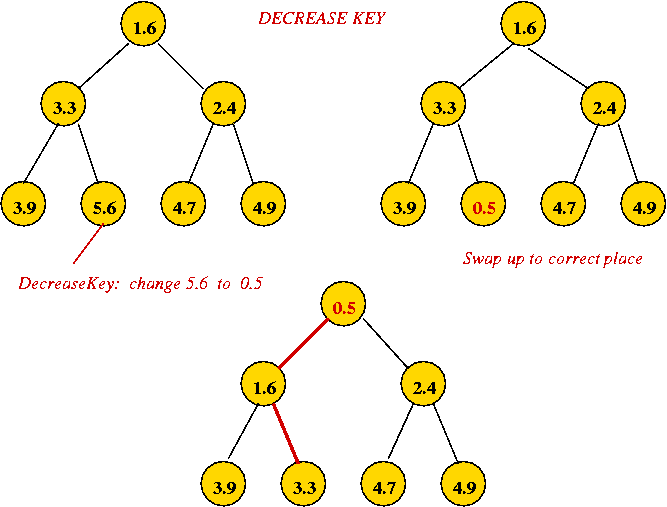• Simply decrease the value and swap it up towards root to correct location.
O(log(n)) worst-case.

Using a priority queue with Prim's algorithm:

```

// Initialize vertex priorities and place in priority queue.
1.   priority[i] = infinity
2.   priorityQueue = all vertices (and their priorities)
// Set the priority of vertex 0 to 0.
3.   decreaseKey (0, 0)

4.   while priorityQueue.notEmpty()
// Extract best vertex.
5.       v = priorityQueue.extractMin()
// Explore edges going out from v.
6.       for each edge e=(v, u) in adjList[v]
7.           w = weight of edge e;
// If there's an edge and it's not a self-loop.
8.           if priority[u] > w
// New priority.
9.               priorityQueue.decreaseKey (u, w)
10.              predecessor[u] = v
11.          endif
12.      endfor
13.  endwhile

// ... build tree in adjacency list form ... (not shown)

Output: Adjacency list representation of MST
```
Analysis:
• Recall: running time of Prim's algorithm depends on n extract-min's and m decrease-key's.
O(n log(n) + m log(n))
= O(m log(n))

## Boruvka's algorithm

It turns out that neither Prim nor Kruskal were the first to address the MST problem [Grah1985]:

• First, note: Kruskal's algorithm was published in 1956, Prim's in 1957.

• A solution to the problem was first published in 1926 by Czech mathematician Otakar Boruvka (1899-1995) [WP-2].

• The problem is itself thought to have been first addressed in 1938 by G.Choquet.

• The essence of Prim's algorithm first appeared in a 1930 paper by V.Jarnik written in Czech.

• Dijkstra re-discovered the algorithm in 1959.
Some books now call this the Jarnik-Prim-Dijkstra algorithm.

• Kruskal and Prim both worked at AT&T Bell Labs, though neither knew of each other until later.

Key ideas in Boruvka's algorithm: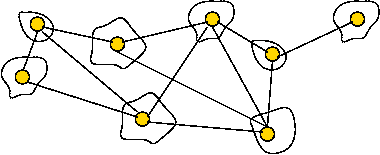• At each Boruvka phase:
• Identify the cheapest edge leaving each tree.
• Merge the trees joined by these edges.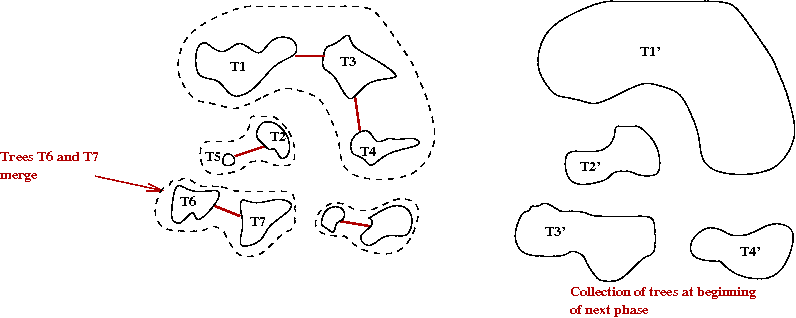• Why does this work?
• The cheapest edge going out from a tree T is the cheapest edge between T and "the outside world"
By the cut-edge property, it must be in the MST.

Boruvka's algorithm with edge contractions:

• What is an edge contraction?
• Merge the two nodes on either side into one new node.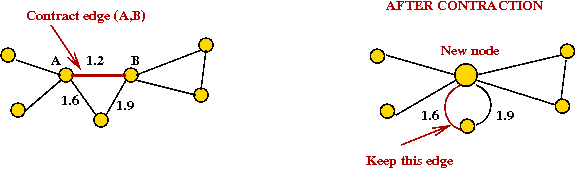• Keep cheapest edge among multiple edges to neighbors.

• The algorithm with contractions:
```
Algorithm: Boruvka (G)
Input: Graph G = (V, E)
1.   Create graph F = (V, φ)     //No edges in F.
2.   while F not connected
3.      for each component in F
4.         add cheapest outgoing edge to MST
5.      endfor
6.      Contract added edges in F and G
7.   endwhile
```

• Example: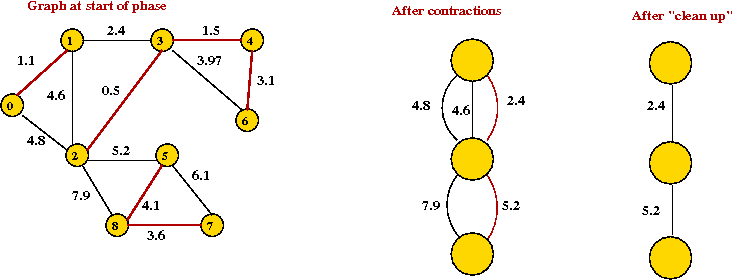Analyis:

• Let Vi be the vertex set after the i-th phase.

• Each contraction reduces the vertex set by at least half
|Vi+1| ≤ ½ |Vi|.
There are at most O(log n) phases.

• In each phase, we examine at most O(m) edges
O(m log(n)) time overall.

• This is as good as Prim+BinaryHeap.

## Enter the data structures, part II: the fibonacci heap

First, the precursor to the fib-heap: the binomial heap [Vuil1978].

• The binomial heap is a radical departure from standard data structures in one way:
Instead of one tree, it uses a collection of trees.

• To avoid confusion, we'll call the whole structure a binomial queue.

• A binomial queue is:
• A collection of binomial trees.
• Each binomial tree in the queue is min-heap-ordered, with nodes that can have more than two children, and has a certain structure (see below).

• Recall that min-heap order means: each parent is "less" than its children.

• A binomial tree Bk of order k is a heap-ordered tree defined recursively: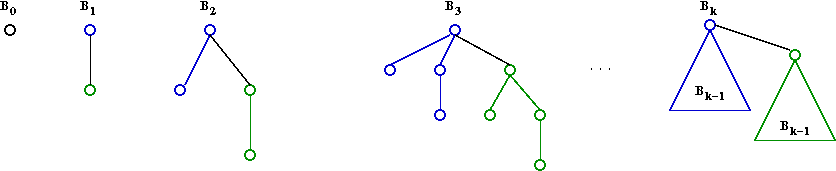• B0 is a node by itself.
• Bk is the tree you get by taking two Bk-1 trees and making one a right child of the other's root.

• A queue can have at most one tree of each order.
e.g., at most one B3 tree.

• The tree merge operation:
• Two Bk trees can be joined as above to make a Bk+1 tree.
• To maintain min-order, the lesser Bk root becomes the Bk+1 root.

• The queue merge operation:
• Two binomial queues can be merged using something like binary addition (with carryover).
• Example: suppose we have two queues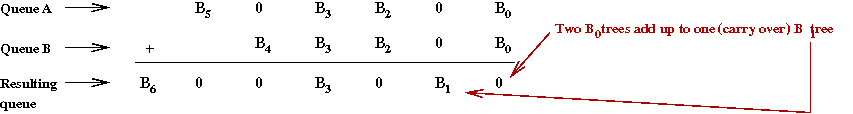• Insert operation:
• Make a new B0 tree and merge that into the existing queue.

• A min pointer:
• The true minimum can be in any one of the trees.
• Keep an external pointer to the running minimum: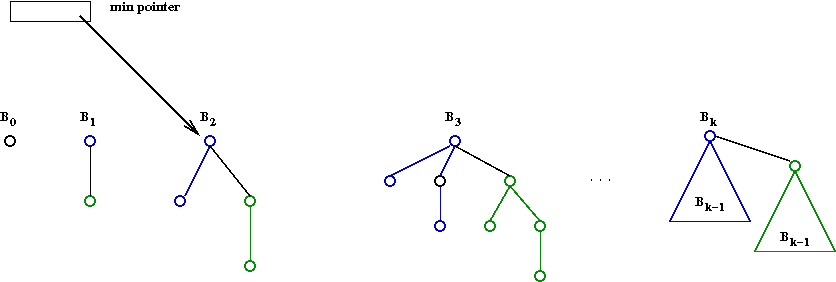• Extract-min operation:
• First, here's another view of a binomial queue (useful for extract-min):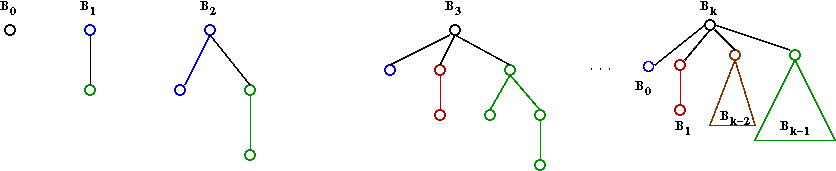• Remove min node (a root of some Bk).
• This will leave child trees B0, B1 ... Bk-1.
• Merge each of these into the rest of the queue.

• Decrease-key operation:
• Decrease value and make heap-adjustments within the tree.
Same as in binary heap.

Exercise: Show that the binomial tree Bk has 2k nodes. Then argue that there are at most O(log n) trees. Binomial queue analysis:

• At first it seems we have the following:
• Insert: O(log n) because of all the "carry" operations that might occur.
• Extract-min: O(log n) because all the subtrees need to be added (a queue-merge operation).
• Decrease-key: O(log n) as in binary heap.

• However, consider this: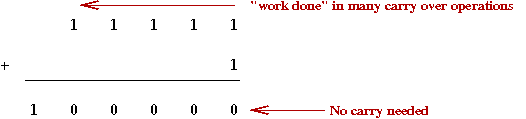• When a carry ripples, it also leaves behind 0's.
Reduces the need for carries in the future.
Over time, the actual number of carries may not be high.

• We want to account for the average cost of an operation over time
Called amortized analysis.
• This is still a worst-case (not average).
• It's really a "worst possible average cost".

• The idea of a potential function:
• In physics, "work done" results in storing potential energy.
• The analog here: "work done" in carries stores "good" zeroes.

• Amortized analysis of insert-operation:
• Let Pi = potential = number of 0's after i-th insert.
• Let Pi - Pi-1 = change in potential.
• Let Ci = true cost of i-th insert.
• Then
Ci = 1 + Pi - Pi-1
i.e., cost = cost-of-new-item  +   ripple-carry
• Hence, over n operations
Σ >Ci = n + Pn - P0
≤ 2n
• Thus, average cost is
(1/n) Σ Ci = O(1).

• Note:
• Above we equated "potential" with "good" zeroes.
• We could just as easily equate "potential" with "bad" ones.
Exactly the same analysis but with change-of-sign for potential.

• Unfortunately: for extract-min and decrease-key, the amortized time is still O(log n).

The Fibonacci heap [Fred1987]: an overview

• The fibonacci heap is a modification of the binomial such that
• Decrease-key takes O(1) amortized.
• Extract min takes O(log n) amortized.

• Again, we switch to calling it a fibonacci queue because whole thing is a collection of trees.

• The fib-queue is based on two ideas:
1. When a decrease-key occurs, don't repair tree.
Instead, "cut out" the subtree where the decrease-key occurs.
2. Dispense with the strict binomial-tree properties:
• Dispense with: at most one Bk tree.
• Dispense with: recursive structure of each Bk.

More detail:

• Define
rank (node) = number of children of the node
rank (tree) = rank (tree's root)

• Maintain a list of trees with min-pointer, as in binomial queue.

• When we merge trees, we will merge two trees of equal rank and create a one-rank-higher tree.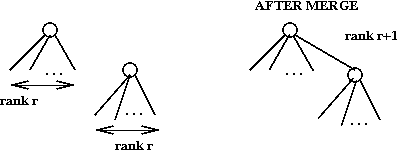• When doing a decrease-key, if heap-order is violated, cut-out node along with subtree: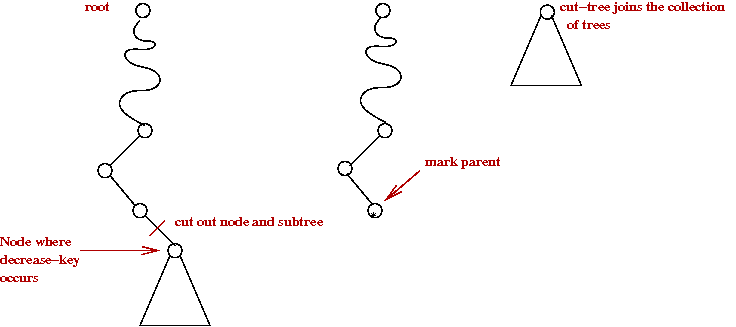• Then, "mark" the parent.
• A parent with two marks is itself cut-out (as a single-node tree):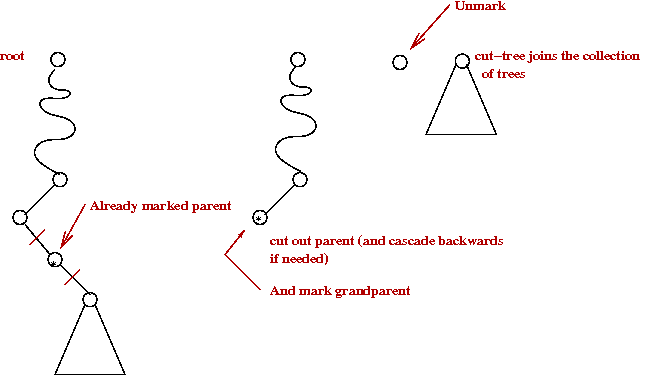• The cutting cascades upwards as long as marked parents need to be cut out.

• Lazy-merge:
• When a cut tree is added to main list, simply concatenate
Do not perform a merge.
• A merge is performed only during extract-min.

• Decrease-key operation:
• As described above: cut out if needed and cascade backwards.
• Note: O(1) time except for cascades.
Will turn out to be O(1) amortized.

• Extract-min operation:
• Remove node as in binomial queue.
• Perform a full merge:
• Start by "adding" the single nodes and work towards adding higher-rank trees.
• Algorithm:
```
for r = 0 to M
while # rank-r trees > 2
merge into a rank r+1 tree
endwhile
endfor
```
• Here, M = the largest rank possible (which is O(log n)).

Exercise: Look up Fibonacci numbers. How are they defined recursively? Prove the following:

• F0 + ... Fn-2 = Fn - 1.
• Fk = O(φk), where φ is the golden ratio.

Analysis:

• First, observe:
• If Ci is the i-th youngest child of a node N, then rank(Ci) ≥ 2
• Proof:
• When Ci is joined with N (during a merge), N had children C1, C2, ... Ci-1.
• Nodes are joined only if they have the same rank.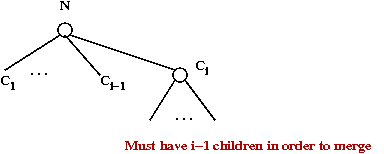• After merging, it can lose children, but at most one
Else, it would itself be cut.
• Thus, it has at least i-2 children.

• Next: a node of rank r has O(Fr) descendants (not just children).
• Let Tr be the smallest tree (fewest nodes) of rank r.
• Then size of T0 = 1 (single node).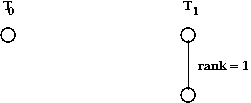|T0| = F1 and |T1| = F2, the second two Fibonacci numbers.

• Now consider a rank r tree and order children by age: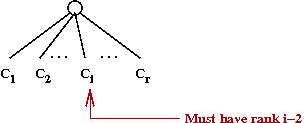• To make the smallest possible tree, assume all children are minimal subtrees.
|Tr| ≥ 1 + |T0| + ... + |Tr-1| = O(Fr).
• But Fr = O(φr).
• Thus, r = O(log|Tr|).
• Thus, with at most n nodes, the rank is at most O(log n).

• Define the "badness" potential as:
potential = #trees + #marked-nodes

• For decrease-key:
• Let C = length of cascade.
• Then, actual cost = C.
• We create O(C) new trees, but reduce marked nodes by O(C).
Total change in potential is O(1).
• Amortized cost: O(1).

• For extract-min:
• Does not change # of marked nodes.
• Total # trees is like the total number of 1's in binary addition.
Same change in potential over a number of operations.
• Total time is bounded by O(log n), the number of trees.
• Amortized cost: O(log n).

• Note: we have glossed over some details in the analysis. See the original paper or the description in [Weis2007].

Back to MST:

• Recall: with Prim's algorithm
• n extract-min's.
• m decrease-key's.

• Thus, for Prim + Fib-heap
O(n log(n) + m) bound.

• Compare with:
• Kruskal: O(m log(n))
Dominated by O(m log(m)) sort.
• Prim + Binary-heap: O(m log(n))
O(n log(n)) extract-min's + O(m log(n)) decrease-key's.
• Boruvka: O(m log(n))
From O(log n) phases, each requiring O(m) edge manipulations.

A simple improvement with Boruvka's algorithm:

• Consider the following algorithm:
Perform O(loglog n) phases of Boruvka (with contractions).
This takes O(m loglog(n)) time.
1. Apply Prim to the resulting graph.
2. Put together all MST edges into single MST.

• Now, we start with n components (the original nodes as one-node trees).
• After phase 1, there are at most n/2 components.
• After phase 2, at most n/22 components.
• ... after phase k, at most n/2k components.

• Thus, if we do O(loglog n) phases, we'll have at most n/log(n) components.
After contraction, this gives a graph with n/log(n) vertices.

• Applying Prim + Fibonacci on the last graph:
• O(m + n/log(n) log(n)) = O(m + n) time.
• Thus, overall: O(m loglog(n)) time.
Fastest so far.

## Fredman and Tarjan's Algorithm

Fredman and Tarjan didn't stop with inventing the Fibonacci heap [Fred1987].

• Consider Prim+Fib again:
O(n log(n)) for n extract-min's and O(m) for m decrease-key's.
• Even if extract-min can't be improved, we can decrease n
Put fewer nodes into the heap.
• Suppose we try to control the size of the heap?
• Recall Boruvka: separate components with their own MST's join together eventually
The heaps of these components could be kept small.
• But we don't know how big these components could get.

• Key ideas: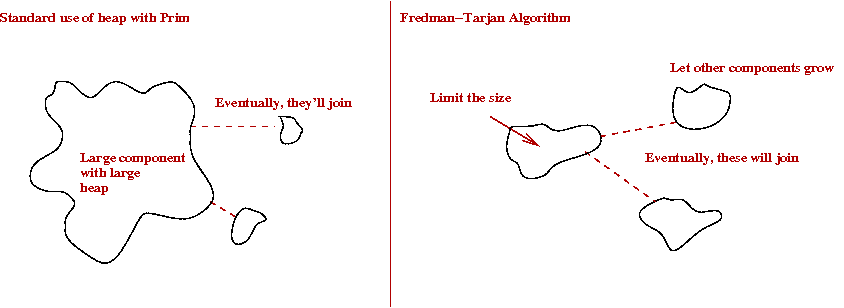• Fix the heap size.
• If a component's heap gets too big, abandon growing the component for now.
• Start another component.

• Fix the heap limit as k (for now).

• We will redefine a Boruvka phase as follows:
```    1.  Grow trees until all heaps reach k-limit.
2.  Contract all trees to get new graph G'
3.  Set G = G' and repeat
```

• Another insight:
• The graphs at successive phases (after contraction) get smaller.
We can use different k values in each phase.
• The more nodes we have, the smaller we should make k.
Because, the more the risk of encountering large heaps.

• Let ni be the number of nodes after (i-1)-st phase (contraction).

• Let ki be the limit for phase i.
Define ki = 22m/ni

• Then, the running time for a single phase takes
O(m + ni log(ki))
= O(m + ni log(22m/ni))
= O(m) .

• Next, we need to bound the number of phases.

• To do this, let's estimate the number of trees in phase i:
• Since we stop trees when heap-size is ki, each tree "touches" ki edges (which are in the heap).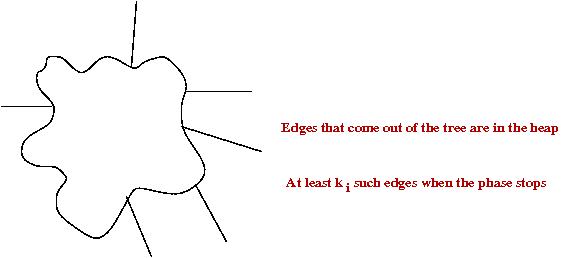• Thus
# trees x ki2m
# trees ≤ 2m / ki
ni+12m / ki (because # trees = # nodes at next level of contraction)
ki+1 = 22m/ni+1 ≥ 2ki.
The ki's are growing.

• The ki's can only grow as large as n.

• Definition: log(i)(n) is i- repeated logs of n: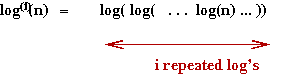• Definition: log*(n) is the number of times you need to take repeated log's to reach 1.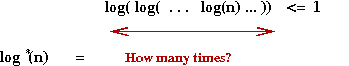• Now, let's work backwards from n.
ki ≥ n
if log(i)ki ≥ log(i)n
which will be true if log(i)2m/ni ≥ log(i)n
which will be true if 2m/ni ≥ log(i)n
which will be true if 2m/n ≥ log(i)n
which will be true if m/n ≥ log(i)n

• Definition: Define β(m,n) = mini log(i)(n) ≤ m/n.

• Thus, there are at most β(m,n) phases.

• Note: β(m,n) ≤ log*(m/n).
So, is this better?
• The comparison to make is:
• Prim+Fib: O(nlog(n) + m).
• Boruvka+Prim+Fib: O(m loglog(n)).
• Fredman-Tarjan: O(m β(m,n)).

• Obviously, Fredman-Tarjan is better than Boruvka+Prim+Fib.

• Clearly, when m < O(n log n), Fredman-Tarjan is better.
• It is possible [Eisn1997] to show that for n>16, m β(m,n) = O(nlog(n) + m).

• Thus, overall, Fredman-Tarjan is the best so far.

## An improvement to Fredman-Tarjan

Gabow et al (including Tarjan) [Gabow1986] found a way to improve the Fredman-Tarjan algorithm:

• Recall that Fredman-Tarjan takes O(mβ(m,n)) time.
• Suppose one could reduce the "work" per phase to O(m/p) for some judiciously chosen p.
• Then, we would have
total work = O(m/p) per-phase x p phases
= O(m) overall.

• Now each phase does a bunch of decrease-keys.
• We are counting this as O(m) decrease-keys.
• Each decrease-key takes O(1) time (Fib-heap)
Can't improve on this.
• Can we reduce the number of decrease-key operations?

• The key idea (in rough):
• Recall: when do we need decrease-key?• Place edges into groups called "packets"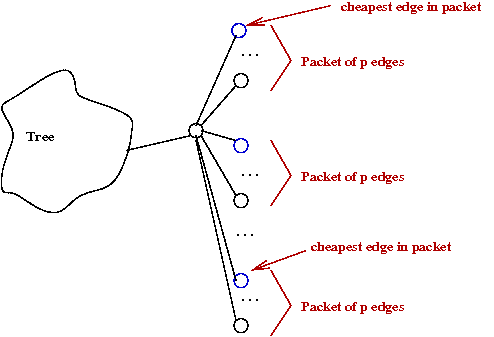• Each packet is partially-sorted once by edge-weight so that cheapest packet is known.
One-time cost of m log(p).
• Apply decrease-key to only "packet leaders."

• Total time: O(mlog(p) + m).

• Choose p=β(m,n).
Total time: O(m log(β(m,n))).

## Numbers big and small

Let's try and get a sense of the size of β(m,n):

• The worst case is when m = O(n2).

• Suppose n = number of atoms in the known universe (estimated).
• n = 1080 = 2266.
m = 2534.
m/n = 2266.
β(m,n) = 9.
logβ(m,n) = 4.

• Thus, logβ(m,n) = 4 for all practical purposes in the running time of O(mlogβ(m,n)).

Exercise: Write down (legibly) the largest number you can think of on a square-inch of paper.

Ackermann's function [Corm2006][WP-3]:

• Consider the function
g(i) = 22    when i=1
g(i) = 2g(i-1)    when i>1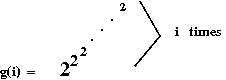• Note:
• log(i)(n) is the inverse of g(i).
• Such a use of repeated exponentiation is called a tetration.

• Clearly, g(i) grows ridiculously fast.

• What if the tetration amount itself grows ridiculously fast?

• Now consider the function h(i) defined as: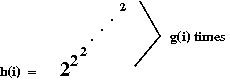• Ackermann's function is similar, even if its definition looks innocuous:
A(1, j) = 2j    for j≥1.
A(i, 1) = A(i-1, 2)    for i≥2.
A(i, j) = A(i-1, A(i,j-1))    otherwise.

• Ackermann (and co-authors) defined this to create an example of a function that is recursive but not primitive-recursive.

• The inverse is defined as follows:
α(m,n) = mini such that A(i, m/n) > log(n).

• To get a sense of its size, A(4,1) > > 1080, the number of atoms in the universe.

• Thus, α(m,n) < 4 for all practical m,n.

• But theoretically, one can show that, for large enough m,n
α(m,n) < β(m,n).

• Thus, an MST algorithm that takes time O(mα(m,n)) would be an improvement over Gabow et al's algorithm.

On the topic of big numbers:

• Consider an integer N and Turing machines with at most n rules.
• Of these, consider those that halt.
• Of those that halt, identify the one that takes the longest
Takes the most steps.

• Define BB(n) = number of steps taken by the longest halting Turing machine with at most n rules.

• Note: BB = "Busy Beaver".

• It is known that:
• BB(1) = 1.
• BB(2) = 6.
• BB(3) = 21.
Laborious construction and checking by hand.
• BB(4) = 107.

• So far, not so impressive.

• But, consider BB(5)
Not known, but it's been shown that BB(5) > 8 x 1015.

## The algorithms of Chazelle and Pettie

History:

• In 1997, Bernard Chazelle [Chaz1997] put together the basic ideas in his new approach to MST's that resulted in an O(m α(m,n)log(α(m,n))) algorithm.

• In 1999, both Chazelle [Chaz2000b] and Seth Pettie [Pett1999] independently improved this to O(m α(m,n)).

• In 2000, Pettie and Ramachandran [Pett2000] showed that, under reasonable assumptions, O(m α(m,n)) is optimal.

Because the algorithms are quite complicated (extreme!), we will only sketch out the key ideas. There are two big innovations:

• A radically new idea for a heap data structure: the soft heap
• Achieves extract-min and decrease-key in O(1) amortized time.
• But insertion takes more time: O(log(1/ε)), where ε is a parameter.
• The radical departure: the heap allows its contents to become corrupted
The value actually changes (increases) and wrong results get reported back.
• At most εn items are corrupted.
• This is exploited in the algorithm to tradeoff speed against the "repair" needed to account for corruption.

• A somewhat radically different approach to using Boruvka-phases.
• In all earlier algorithms: Boruvka phases were used (sometimes with size-parameters) to build the tree bottom-up.
The contractions result in a hiearchy of components
• The "contraction tree" was useful in analysis, but not used in the algorithm.
• Here, instead, a "contraction tree" is first constructed according to pre-specified size constraints.
• The actual MST construction uses the tree.
The tree is used to guide the MST-building process.
• MST construction itself occurs in separate phases.

Key ideas:

• Part I: building the contraction tree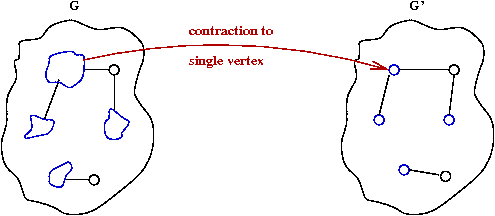```
1.   G = original graph
2.   while G not a single vertex
3.      Identify sub-graphs according to size parameters
4.      for each subgraph
6.         Create node in contraction tree
7.         Place sub-graph nodes as children
8.         Contract into single vertex of G'
9.      endfor
10.     G = G'
11.  endwhile
12   return contraction tree
```
This results in the contraction tree: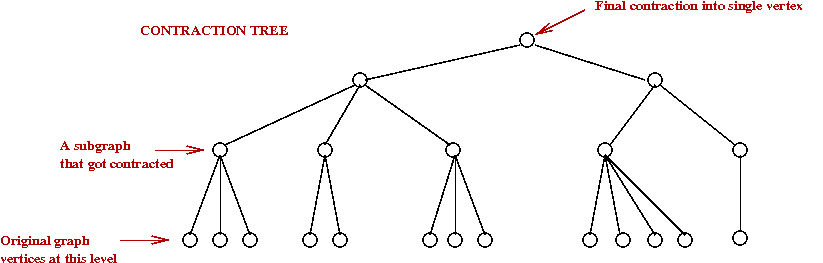• Note:
• Along the way (above), identify so-called "bad" edges.
These are edges whose corrupted values might affect the MST.
• Turns out, a bottom-up construction takes too long
The tree is built in post-order to limit the number of "active" sub-graphs at any time to the tree-height.
• During construction, some edge weights in the soft-heaps get corrupted.
• Some corrupted edges don't matter to the MST.
Those that do are called "bad".

• Part 2: Recurse down the tree and build an MST using non-bad edges.
• This is done in using Boruvka-phases, but limited by the already-chosen sub-graphs and contractions.

• Part 3: Add back the bad edges (with their original costs) and recurse again.

Analysis:

• The height and sub-graph sizes of the contraction tree are under our control (as in Fredman-Tarjan).

• By choosing these carefully, the O(mlogα(m,n)) time-bound is achieved.

Optimality:

• It can be shown that the above algorithm takes O(m) time if m/n > O(log(3)(n))
i.e., it fails O(m) only for extremely-sparse graphs (almost tree-like)

• Compute MST's of all possible graphs with r vertices.
• Store these in a pre-computed look-up table.
• There are at most 2r2 such graphs.
• If r can be kept small, one can exploit this for optimality.
Note: r = O(log(3)(n)).

• How?
• Contract graph to reduce #vertices by factor of O(log(3)(n)).
Desired density is achieved.
• Pick sub-graphs whose optimality can be immediately determined from lookup table.
No cost to finding MST of these subgraphs.
• This increases the edge density to above O(log(3)(n)).
• Now apply O(m) algorithm to remainder and join MST's.

1. How find all possible r-sized subgraphs with weights?
Not the same as all possible r x r binary matrices.
2. How to make sure that this takes reasonable time?

• Problem 1: solve using decision-tree approach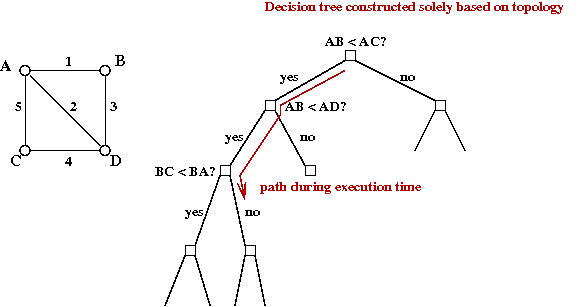• Instead of "all possible sub-graphs", compute all possible "decision trees on such sub-graphs".
• This is a well-known approach (in the theory world).

• Problem 2: not solved.
• Decision-tree construction time can be bound.
• But execution time depends on actual values.
Execution-time can't be known (or tightly bound) ahead of time.
• But, it can be shown, that it's optimal (in execution time).
Resulting algorithm is optimal (even if we can't pin down the running time).

## Summary

Let's step back and look at the history one more time:

• Otakar Boruvka's algorithm in 1926.
O(m log(n)) time (although he probably didn't know it).
From O(log n) phases, each requiring O(m) edge manipulations.

• Jarnik in 1930: essentially Prim's algorithm
O(n2) because they didn't know of heaps at that time.

• Kruskal in 1956: O(m log(n))
Dominated by O(m log(m)) = O(m log(n)) sort.

• Prim in 1957: re-discovered Jarnik's algorithm
Still O(n2).

• Dijkstra in 1959: re-discovered Jarnik-Prim algorithm.

• The use of binary heaps: (1960's?)
• Prim + binary-heap: O(m log(n))
O(n log(n)) extract-min's + O(m log(n)) decrease-key's.
No faster than Boruvka's original algorithm (analysed in modern times).

• Improvement's to Boruvka, independently by Yao, and Cheriton-Tarjan in 1975-76.
O(m loglog(n))

• Binomial heaps in 1978.

• 10 year gap.

• Fredman-Tarjan's Fibonacci heap in 1987:
Direct improvement of Prim's algorithm to O(nlog(n) + m).

• Fredman-Tarjan algorithm in 1987: control heap growth
O(mβ(m,n)).

• Gabow et al's improvement soon after:
O(m logβ(m,n)).

• 10 year gap.

• Chazelle's soft-heap approach in 1997
O(m α(m,n)logα(m,n)).

• Chazelle's and Pettie's improvements to Chazelle's approach in 1999:
O(m α(m,n)).

• Pettie and Ramachandran's decision-tree algorithm and proof of optimality in 2000.
Exact time unknown but at most O(m α(m,n)).

End of story? Various other interesting variations

• An O(m) randomized algorithm by Karger et al. in 1995.

• Simpler versions of the soft heap, e.g., Kaplan et al in 2009.

• The run-relaxed heap by Driscoll et al (which improves decrease-key to O(1) worst-case).

• Faster (O(n logn) algorithms for Euclidean MST's.

[WP-1] Wikipedia entry on Minimum Spanning Trees.
[WP-2] Wikipedia entry on Otakar Boruvka.
[WP-3] Wikipedia entry on Ackermann's function.
[Aaro] S.Aaronson. Who can name the biggest number?
[Boru1926] O.Boruvka. (paper written in Czech).
[Chaz1997] B.Chazelle. A faster deterministic algorithm for minimum spanning trees. Symp. Foundations of Computer Science, 1997, pp.22-31.
[Chaz2000] B.Chazelle. The soft heap: an approximate priority queue with optimal error rate. J. ACM, 47:6, 1012-1027, Nov. 2000.
[Chaz2000b] B.Chazelle. A Minimum Spanning Tree Algorithm with Inverse-Ackermann Type Complexity. J. ACM, 47, 2000, pp. 1028-1047.
[Cher1976] D.Cheriton and R.E.Tarjan. Finding minimum spanning trees. SIAM J.Computing, Vol. 5, 1976, pp.724-742.
[Corm2006] T.H.Cormen, C.E.Leiserson, R.Rivest and C.Stein. Introduction to Algorithms (2nd Edition), McGraw-Hill, 2006.
[Dixo1992] B.Dixon, M.Rauch and R.E.Tarjan. Verification and sensitivity analysis of minimum spanning trees in linear time. SIAM J.Computing, 21:6, 1992, pp.1184-1192.
[Dris1988] J.R.Driscoll, H.N.Gabow, R.Shrairman and R.E.Tarjan. Relaxed heaps: an alternative to Fibonacci heaps with applications to parallel computation. Comm.ACM, 31:11, 1988, pp.1343-54.
[Eisn1997] J.Eisner. State-of-the-art algorithms for minimum spanning trees: A tutorial discussion. Manuscript, University of Pennsylvania, April 1997.
[Fred1987] M.Fredman and R.Tarjan. Fibonacci heaps and their uses in improved network optimization algorithms. J. ACM, 34, 1987, pp.596-615.
[Gabo1986] H.N.Gabow, Z.Galil, T.Spencer and R.E.Tarjan. Efficient algorithms for finding minimum spanning trees in undirected and directed graphs. Combinatorica, 6:2, 1986, pp.109-122.
[Grah1985] R.L.Graham and P.Hell. On the history of the minimum spanning tree problem. Annals of the History of Computing, 7, 1985, pp.43-57.
[Jarn1930] V.Jarnik. (paper written in Czech).
[Kapl2009] H.Kaplan and U.Zwick. A simpler implementation and analysis of Chazelle's soft heaps. In Proc. ACM -SIAM Symposium on Discrete Algorithms (SODA), New York, NY, Jan 2009, pp. 477-485.
[Karg1995] D.R.Karger, P.N.Klein and R.E.Tarjan. A randomized linear-time algorithm to find minimum spanning trees. J. ACM, 42:2, March 1995, pp.321-328.
[King1995] V.King. A simpler minimum spanning tree verification algorithm. Proc. Int. Workshop on Algorithms and Data Structures, 1995, pp.440-448.
[Krus1956] J.B.Kruskal. On the shortest spanning subtree of a graph and the traveling salesman problem. Proc. Am. Math. Soc., 7, 1956, pp.48-50.
[Krus1997] J.B.Kruskal. A reminiscence about shortest spanning trees. Archivum Mathematicum, 1997, pp.13-14.
[Pett1999] S.Pettie. Finding Minimum Spanning Trees in O(m alpha(m,n)) Time. Technical report TR-99-23, University of Texas, Austin, 1999.
[Pett2000] S.Pettie and V.Ramachandran. An Optimal Minimum Spanning Tree Algorithm. J. ACM, 49:1, 2000, pp. 16-34.
[Prim1957] R.C.Prim. Shortest connection networks and some generalizations. Bell Sys. Tech. J., 36, 1957, pp.362-391.
[CS153] R.Simha. Course notes for CS-153 (Undergraduate algorithms course).
[Weis2007] M.A.Weiss. Data Structures and Algorithm Analysis in Java, 2nd Ed., Addison-Wesley, 2007.
[Vuil1978] J.Vuillemin. A data structure for manipulating priority queues. Comm. ACM, 21:4, April 1978, pp. 309-315.
[Yao1975] A.Yao. An O(E loglog(V)) algorithm for finding minimum spanning trees. Inf.Proc.Lett., Vol. 4, 1975, pp.21-23.